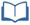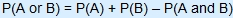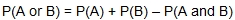# Teacher Resources

## Fan Appreciation Night, Part 2

On this page you will find lesson objectives, standards, instructional notes and UDL opportunities.

Estimated Time:  30 - 40 minutes

###Lesson Objectives:

• Students will distinguish between mutually exclusive and mutually inclusive events.
• Students will find probabilities of compound events involving unions P(A or B) and appropriately use the Addition Rule.

Key Common Core State Standards:

S.CP.1 Describe events as subsets of a sample space (the set of outcomes) using characteristics (or categories) of the outcomes, or as unions, intersections, or complements of other events (“or,” “and,” “not”).

S.CP.2 Understand that two events A and B are independent if the probability of A and B occurring together is the product of their probabilities, and use this characterization to determine if they are independent.

S.CP.7 Apply the Addition Rule,, and interpret the answer in terms of the model.

Supporting Common Core State Standards:

7.SP.5 Understand that the probability of a chance event is a number between 0 and 1 that expresses the likelihood of the event occurring. Larger numbers indicate greater likelihood. A probability near 0 indicates an unlikely event, a probability around 1/2 indicates an event that is neither unlikely nor likely, and a probability near 1 indicates a likely event.

7.SP.6 Approximate the probability of a chance event by collecting data on the chance process that produces it and observing its long-run relative frequency, and predict the approximate relative frequency given the probability.

7.SP.7 Develop a probability model and use it to find probabilities of events. Compare probabilities from a model to observed frequencies; if the agreement is not good, explain possible sources of the discrepancy.

1. Develop a uniform probability model by assigning equal probability to all outcomes, and use the model to determine probabilities of events.
2. Develop a probability model (which may not be uniform) by observing frequencies in data generated from a chance process.

7.SP.8 Find probabilities of compound events using organized lists, tables, tree diagrams, and simulation.

1. Understand that, just as with simple events, the probability of a compound event is the fraction of outcomes in the sample space for which the compound event occurs.
2. Represent sample spaces for compound events using methods such as organized lists, tables and tree diagrams. For an event described in everyday language (e.g., “rolling double sixes”), identify the outcomes in the sample space which compose the event.
3. Design and use a simulation to generate frequencies for compound events.

Standards for Mathematical Practice Emphasized:

MP.1 Make sense of problems and persevere in solving them.
MP.2 Reason abstractly and quantitatively.
MP.3 Construct viable arguments and critique the reasoning of others.
MP.5 Use appropriate tools strategically.
MP.6 Attend to precision.
MP.7 Look for and make use of structure.

## Instructional Notes:

In this lesson, students will distinguish between mutually exclusive and mutually inclusive events.  Students will calculate.  This lesson will include opportunities for the student to apply the Addition Rule.   This task will be a continuation of the “Fan Appreciation Night” (but the current task is independent of this previous task).

> Go to Fan Appreciation Night, Part 2 lesson

## Sample Responses

For sample responses to the Algebra II Journal questions, visit the Algebra II Journal in the Teacher Resources.

## Fan Appreciation Night, Part 2 - Page 4

Venn diagrams can be powerful tools to help students distinguish between mutually exclusive (disjoint) events and mutually inclusive events.

> Go to lesson, page 4

## Fan Appreciation Night, Part 2 - Page 7

As an optional extension or in-class activity, have students create their own criteria for winning two prizes.  Have students calculate the probability of winning one item or the other.  Students could present their proposals and probabilities to the class.

> Go to lesson, page 7

## UDL Opportunities:

Checkpoint 2.1 Clarify vocabulary and symbols.
Checkpoint 2.3 Support decoding of text, mathematical notation, and symbols.
The Student Resources section includes key vocabulary clarifications that will support the decoding of text and mathematical language.  It may be helpful to introduce some of the key terms prior to the start of this lesson.

Checkpoint 3.2:  Highlight patterns, critical features, big ideas, and relationships.
Checkpoint 3.3 Guide information processing, visualization, and manipulation.
Checkpoint 3.4:  Maximize transfer and generalization.
By the end of this lesson, students should be able to distinguish between mutually inclusive and mutually exclusive events and calculate probabilities of compound events that involve unions, i.e. P(A or B).

Checkpoint 6.2:  Support planning and strategy development.
Checkpoint 7.2:  Optimize relevance, value, and authenticity.
Checkpoint 8.3:  Foster collaboration and community.
Checkpoint 9.3 Develop self-assessment and reflection.

The activities in this lesson are based on real-world contexts to increase relevance and student interest.  Consider having students work in groups to generate additional scenarios for winning prizes at the game.